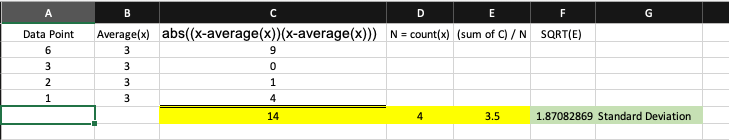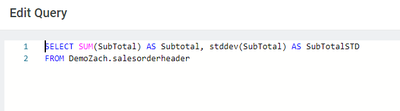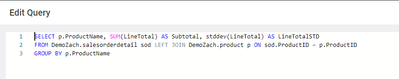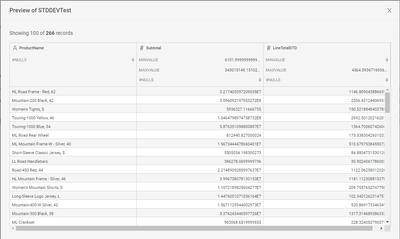cancel
Showing results for
Did you mean:

## Standard DeviationPartner

Hi,

=IFERROR(STDEV.P(BQ14348:CB14348)/AVERAGE(BQ14348:CB14348),0)

Could please let me know how to convert above "STDEV.P" excel function in Incorta.

4 REPLIES 4Employee

KHK,

Unfortunately, no we do not have a built in function for this.  We have many of the functions that in aggregate support the calculation such as ABS(), AVERAGE() and SQRT(). In a multi pass process it would look something like:

1. for each data point x calculate distance from mean, squared: abs((x-avg(x))(x-avg(x)))
2. sum each of the above, and divide by count(x)
3. sqrt(#2)

However, the easier way to do this is by using an python MV and using a python dataframe function to calculate the STDEV from the elements in the dataframe.  Pandas supports something like

`df['amount'].std()`EmployeeRocketeer

Hello JeffW,

I'm working with a dataset where the grouping criteria are determined by specific requirements and are not fixed or limited.

Typically, other aggregate functions operate on the columns designated in the grouping context. Is it possible to apply the stddev() function in a manner similar to other aggregate functions such as sum and average, considering this dynamic grouping?Employee

Adding on to Jeffs approach, if you happen to be more SQL oriented you can alternatively use SparkSQL or Postgres SQL 'STDDEV' Function within a Materialized View.Output:Similarly, if we want to show the standard deviation grouped by product in the same scenario here is a simple example.

With Group By:Output:Regards,

Zach